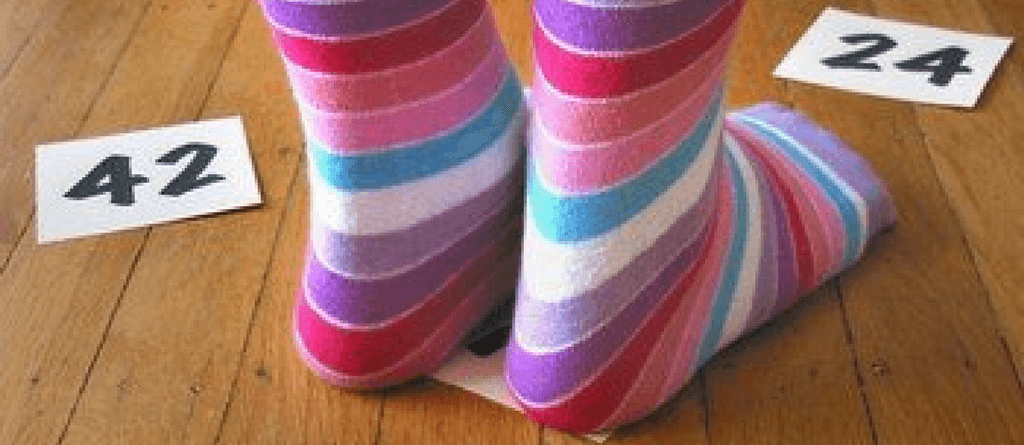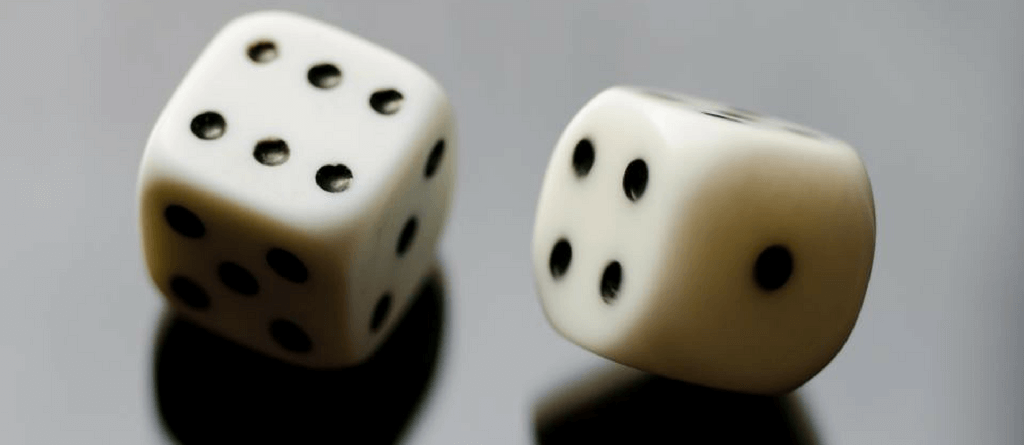Subtraction has always been one of the most confusing concepts of elementary school math. Kids avoiding subtraction practice is a very normal scene. Single digit subtraction is comparatively easy. Enter double digit subtraction and subtraction with regrouping, and you will find kids running away from math. It is important to play with subtraction at an early age when the concept is being introduced to child. Simple math activities and games help in doing so. This way, they will want to practice more.

Here we give you 3 subtraction games for kids with which you can make them addicted to subtraction practice. These are simple math facts games and are lots of fun. What more? Even though these are subtraction games, they can be easily modified into math games of both addition and subtraction or even later into multiplication games.

## Skills kids will practice with these subtraction games

The below given three subtraction games ensureÂ a lot of subtraction practice for your kids. Following are few key skills that kids can master through these games:

1. Single digit subtraction
2. Single digit/ Double digit subtraction
3. Subtraction with regrouping

### SUBTRACTION GRID MATH ACTIVITYThis is one of those subtraction games which you and your kids can enjoy outdoors. But in case you have sufficient space, one can play it indoors too.

#### What do you need?

• Blackboard Chalks
• Measuring Tape/ruler
• 2 Bean Pouches/pebble pouches

#### How to play?

1. Find a wide open space like a driveway or a backyard or a cemented court.
2. Draw a 5 x 5 grid on the ground with the help of the chalk. Make sure that each square in the grid is at least 1 foot wide and long. Use the measuring tape in order to get the right square sizes.
3. Fill the squares with random numbers. The numbers should be big and bold enough for clear visibility.
4. Move 4 – 6 feet away from the grid and draw another line parallel to the grid using the chalk. This will be the ‘Throw Line’.
5. Ask your kid to stand on the Throw Line; hand her 2 Bean Pouches and ask her to throw them on the grid. After throwing, she will have to identify the higher number and the lower number and then subtract the 2. The answer to that will be her score for the round.
6. In case 2 or more players are playing, play 5-6 (or more) rounds and add the individual scores of all the rounds. The player with the highest score wins the game.

#### Few things to note

• Remember that the numbers on the grid should be apt for the level of subtraction practice your kids need. For younger kids fill the grid with single and double digit numbers and for older kids fill it with higher values.
• Do not group all the large numbers or all the small numbers together on the grid. Spread them fairly across the grid.
• You can increase or decrease the distance between the grid and the Throw Line as per the throwing convenience of kids.

Get an extra practice through math worksheets. These subtraction worksheets are colorful and fun. Each worksheet has a story that children can connect with and solve.

### SUBTRACTION TWISTERÂ MATH ACTIVITYSubtraction twister is a unique one among the many subtraction games. This game combines math with music. The best part of this subtraction game is that through it kids will actually end up completing their subtraction facts homework. Once again, this is one of those subtraction games which requires substantial space. So according to the availability, it can be conducted either indoors or outdoors.

#### What do you need?

• Thin Cardboard Sheet/ Thick sheet/Chart paper
• Thick Markers/Sharpies
• Sticky Tape
• Music Player

#### How to Play?

1. Go through the subtraction facts homework assigned to your kid and list out the answers to all the problems.
2. Next, cut the cardboard sheet into 10-inch squares. Cut as many squares as there are subtraction facts problems.
3. Take the thick marker and write the answers on the cardboard squares. Make sure that the numbers are big enough to cover a large space of the square and bold enough to be visible from a few feet away.
4. With the help of the sticky tape, stick the cardboard squares, face up and randomly across the play area.
5. Turn on the music and ask your kid to dance on top of the numbers. Pause the music after a few seconds, and loudly call out one of the subtraction facts problems like “nine minus four” OR “fifty-nine minus thirty-six”.
7. For every correct answer, your kid scores 10 points and for incorrect ones, she gets a -5.

### FINAL COUNTDOWNÂ MATH ACTIVITYThis subtraction game is to be played indoors. Although it can be played individually, but the real fun lies in playing it with two or more players. Also, there are two versions of this game – single dice game and double-dice game.

#### What do you need?

• Blank Papers
• 1 Pen per Player
• 2 Dice
##### Single Dice

The single dice game is apt for kids who have just started with subtraction. In this game, kids will subtract single digit numbers from double-digitÂ numbers and single-digit numbers.

1. Write the number 1-20, in descending order, vertically on the sheet of paper. There should be as many columns of 1-20 as the number of players.
2. Ask each player to roll the dice once. The player who rolls the highest number becomes the first player. The game starts with the first player rolling the dice.
3. The game starts at the number 20. The players, in their respective turns, will roll the dice, subtract the rolled number from 20 to find her new position. Say, player 1 rolls a 5, she will subtract 5 from 20 and circle 15. That is her new position.
4. The game continues in a similar manner. The player who reaches number 1 first wins the game.
##### Double Dice

This is a good game for double digit subtraction practice. Through the double dice game kids even get a chance to practice subtraction with regrouping.

1. For this game, hand over one blank paper to each player and ask them to write 100 on top of the sheet.
2. Next, the first player will roll the two dice, add the two numbers on the dice, subtract the answer from 100, and write the result below 100. Say, she rolled a 6 and 4 on the two dice. She will add 6 and 4, and then subtract the answer i.e. 10 from 100, and write 90 below 100.
3. Similarly, each player will take turns, roll the dice, add the numbers, subtract them from 100, and write the result below 100.
4. In the next round, the players will follow the same steps and subtract from the result of the previous round. Say, for this round, player 1 rolls a 2 and 4. She will add 2 and 4 and deduct the answer i.e. 6 from 90 and write 84 below 90.
5. The game goes on in a similar manner. The player who reaches Zero first wins the game.
##### Variations
• Single-Dice Game: Create columns of 1-50 or the range of numbers for which your kids need subtraction practice.
• Double-Dice Game: To increase the difficulty increase the range of numbers beyond 100 and play with 3 dice.